Next: Representation of Waves via Up: Wave Mechanics Previous: Photoelectric Effect

# Electron Diffraction

In 1927, George Paget Thomson discovered that if a beam of electrons is made to pass through a thin metal film then the regular atomic array in the metal acts as a sort of diffraction grating, so that when a photographic film, placed behind the metal, is developed, an interference pattern is discernible. This implies that electrons have wave-like properties. Moreover, the electron wavelength,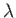, or, alternatively, the wavenumber,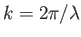, can be deduced from the spacing of the maxima in the interference pattern. Thomson found that the momentum,, of an electron is related to its wavenumber,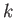, according to the following simple relation: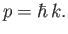(C.3)

The associated wavelength,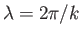, is known as the de Broglie wavelength, because this relation was first hypothesized by Louis de Broglie in 1926. In the following, we shall assume that Equation (C.3) is a general result that applies to all particles, not just electrons.

It turns out that wave-particle duality only manifests itself on lengthscales less than, or of order, the de Broglie wavelength. Under normal circumstances, this wavelength is fairly small. For instance, the de Broglie wavelength of an electron is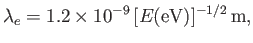(C.4)

where the electron energy is conveniently measured in units of electron-volts (eV). (An electron accelerated from rest through a potential difference of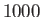V acquires an energy ofeV, and so on. Electrons in atoms typically have energies in the range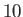to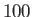eV.) Moreover, the de Broglie wavelength of a proton is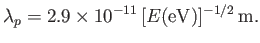(C.5)Next: Representation of Waves via Up: Wave Mechanics Previous: Photoelectric Effect
Richard Fitzpatrick 2016-01-25